# Difference between revisions of "SOCR EduMaterials Activities ScatterChart"

## Summary

This activity describes the need, general methods and SOCR utilities for scatter plot.

## Goals

The purposes of this activity are to:

• illustrate the use of scatter plots
• show the important of them
• allow users to use SOCR scatter plots for their educational advantage

## Background

Similar to Line Graphs, Scatter Charts are a simple visualization for data points to be plotted without drawn lines connecting them. They are often useful for comparing each point separately or groups of points from different events. Characteristics and behaviors of these data sets may be determined by observing Scatter Charts.

For references, visit Scatter Charts and More Examples.

## Description

Go to the SOCR Charts and select Line Charts from the items located on the left, then select the demonstration for Scatter Charts: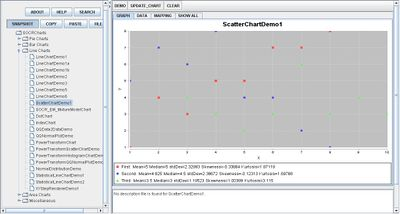The image above is a demonstration of a simple Scatter Chart. Note that below the graph are shown the statistical values for each event.

## Examples & Exercises

• Exercise 1: Suppose you are given a set of data points based on the score of each student during the final. Create a scatter plot of the given information. What can be drawn from this set of values? Can the minimum, maximum, and average score be found from the scatter plot?
• Exercise 2: If you are given the average price of gasoline across the nation, can a scatter plot be made to show the gathered raw information?
• Exercise 3: Is the scatter plot still useful for data information with less than 5 values? How about for values within a very small range?

## Data Type and Format

By clicking Data between the Graph and Mapping button, it allows users to input or vary the values of the data set. There is one type of variable which is quantitative or numerical that is listed to the corresponding event: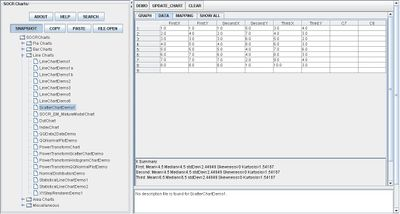## Example (Human Heights and Weights)

Generate a scatter plot of the first 20 pairs of the Human Height and Weight data. Copy the data with your mouse.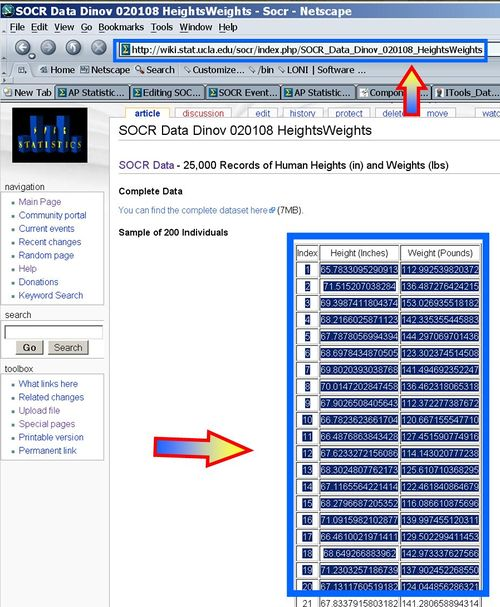Paste it in the SOCR Scatter Plot Chart (you need to enter an additional column for the index of the weight).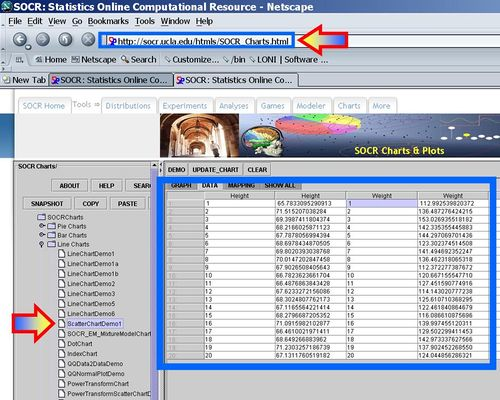You should get a Scatter plot like this. Notice the summary statistics on the bottom!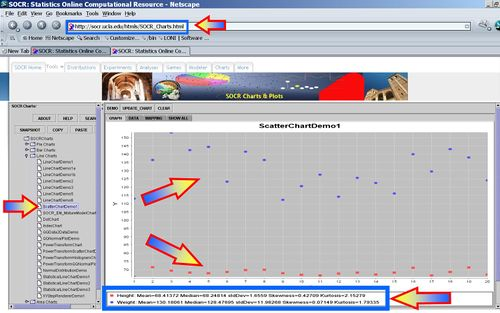You can also do a Scatter plot of Weight agains Height to explore the linearity of their relation.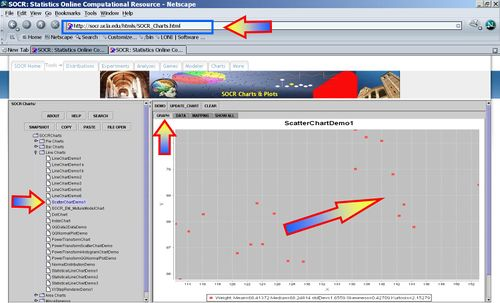## Applications

One of the most persuasive elements when proposing data and literature to others is a well-designed chart presentation.

For example, students in labs may want to present their table of values in a graph. By using the Scatter Chart, every unique data value will be equally represented in the Scatter Chart.

Suppose that researchers are interested in the cholesterol level in a group of adults. By plotting every data set, all of the values are equally represented in the Scatter Chart.